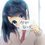While going through inequality books, I've found a very interesting problem:

Given that $a$, $b$, $c$ are positive real numbers such that $a^2+b^2+c^2+2abc=1$. Prove that: $a^2b^2+b^2c^2+c^2a^2 \ge 12a^2b^2c^2$.

I've done a few:

$a^2b^2+b^2c^2+c^2a^2 \ge 12a^2b^2c^2$ $\Longleftrightarrow \frac { 1 }{ a^{ 2 } } +\dfrac { 1 }{ b^ 2 } +\frac { 1 }{ c^ 2 } \ge12$

This can be proved if : $\dfrac{9}{a^2+b^2+c^2} \ge 12$ $\Longleftrightarrow a^2 + b^2 + c^2 \le \dfrac{3}{4}$

This is where I've stucked, since I cannot think of any connection between it and the fact that $a^2+b^2+c^2+2abc=1$.Note by Trung Đặng Đoàn Đức
5 years, 1 month ago

This discussion board is a place to discuss our Daily Challenges and the math and science related to those challenges. Explanations are more than just a solution — they should explain the steps and thinking strategies that you used to obtain the solution. Comments should further the discussion of math and science.

When posting on Brilliant:

• Use the emojis to react to an explanation, whether you're congratulating a job well done , or just really confused .
• Ask specific questions about the challenge or the steps in somebody's explanation. Well-posed questions can add a lot to the discussion, but posting "I don't understand!" doesn't help anyone.
• Try to contribute something new to the discussion, whether it is an extension, generalization or other idea related to the challenge.

MarkdownAppears as
*italics* or _italics_ italics
**bold** or __bold__ bold
- bulleted- list
• bulleted
• list
1. numbered2. list
1. numbered
2. list
Note: you must add a full line of space before and after lists for them to show up correctly
paragraph 1paragraph 2

paragraph 1

paragraph 2

[example link](https://brilliant.org)example link
> This is a quote
This is a quote
    # I indented these lines
# 4 spaces, and now they show
# up as a code block.

print "hello world"
# I indented these lines
# 4 spaces, and now they show
# up as a code block.

print "hello world"
MathAppears as
Remember to wrap math in $$ ... $$ or $ ... $ to ensure proper formatting.
2 \times 3 $2 \times 3$
2^{34} $2^{34}$
a_{i-1} $a_{i-1}$
\frac{2}{3} $\frac{2}{3}$
\sqrt{2} $\sqrt{2}$
\sum_{i=1}^3 $\sum_{i=1}^3$
\sin \theta $\sin \theta$
\boxed{123} $\boxed{123}$

Sort by:

Well, one thing I would like to suggest is that never try to reverse-engineer an inequality. Most of times you'll end up with something incorrect. As you can see here - you pose that $a^2+b^2+c^2 \leq \frac{3}{4}$ but infact, the opposite inequality holds true.

EDIT : Although sometimes, you might end up with a trivial solution for an inequality after reverse-engineering it.

Anyways, here's the solution.

First, let's try to deduce some results from the given expression. We have-

$a^2+b^2+c^2 = 1-2abc$

Using AM-GM inequality we get that

$a^2+b^2+c^2 \geq 3(abc)^{2/3}$

$\Rightarrow (1-2abc)^3 \geq 27(abc)^2$

Substituting $u=abc$, then we have

$(1-2u)^3 = 1-8u^3-6u+12u^2 \geq 27u^2$ $\Rightarrow 8u^3+15u^2+6u-1 \leq 0$

Let $f(u) = 8u^3+15u^2+6u-1$. It's easy to see that $f(-1)=0$. Thus, after factorizing out $u+1$, we are left with $f(u)=(u+1)(8u^2+7u-1)$. And hence $f(u)=(u+1)^2(8u-1)$.

But we need to find solutions for $f(u)\leq 0 \Rightarrow (u+1)^2(8u-1) \leq 0$.

This gives us $u \leq \frac{1}{8}$. And thus,

$\boxed{abc \leq \frac{1}{8}}$

Now, let's try to implement this result. Observe that, by AM-GM inequality we have

$\frac{1}{a^2}+\frac{1}{b^2}+\frac{1}{c^2} \geq \frac{3}{(abc)^{2/3}} \geq 3(8)^{2/3} \geq 12$

On rearranging we get our desired result

$a^2b^2+b^2c^2+c^2a^2 \geq 12a^2b^2c^2$

P.S. - Since $abc \leq \frac{1}{8}$, we have $a^2+b^2+c^2 \geq 1-2\left(\frac{1}{8}\right) = \frac{3}{4}$

- 5 years, 1 month ago

Thanks :) Learned some tips too :v

By the way, can you give me some tricks or advices to solve inequalities? I usually do reverse-engineering but I've completely changed my mind after this :)

- 5 years, 1 month ago

Ok, first thing to keep in mind is that you should be familiar with the very basic inequalities like

• AM-GM Inequality
• Cauchy-Schwarz Inequality
• T2's Lemma
• Power-Mean Inequality
• Rearrangement Inequality
• Jensen's Inequality
• Schur's Inequality

(I hope I am not missing out any important ones)

Then must try out examples as many as you can. Here are few to start with -

1. If $a,b,c \in \mathbb{R}^+$, prove that $\frac{a}{b+c}+\frac{b}{c+a}+\frac{c}{a+b} \geq \frac{3}{2}$

2. If $a$ and $b$ are positive real numbers such that $a+b=1$. Prove that $a^ab^b+a^bb^a \leq 1$

3. Let $a,b,c$ be positive reals such that $\frac{1}{a^2+b^2+1}+\frac{1}{b^2+c^2+1}+\frac{1}{c^2+a^2+1} \geq 1$. Prove that $ab+bc+ca \leq 3$

4. Prove the AM-GM Inequality

BONUS : Try to prove inequality #1 with as many methods as you can.

- 5 years, 1 month ago

Very curious to know the answer but too tuff if you find answers post I will also try

- 5 years, 1 month ago